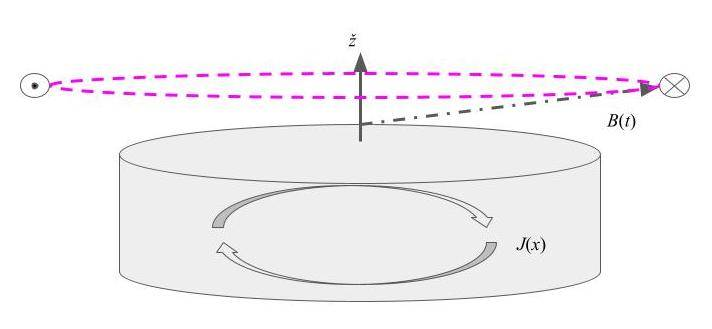# Perfect conductor in rotating magnetic field

• I
johnfulgor
Hello all,
what would happen to a perfectly conducting cylinder immersed in a rotating magnetic field, with the rotation axis parallel to that of the cylinder? I guess the cylinder would start to rotate with the field? Right?
Thank you

Gold Member
The cylinder is perfectly conducting. Why should the cylinder move when the electrons can re-arrange themselves freely? I haven't solved it analytically, but my sense is that the magnetic field will exert a force on the electrons but the electrons will not exert a force on the conducting cylinder. In other words, the magnetization of the cylinder will rotate with the magnetic field but the cylinder itself will not rotate.

•osilmag
Gold Member
Do you have a visual? If the magnetic field is the same strength all around the cylinder and the cylinder is solid what Twigg said is true. One of Maxwell's equations states this.

Last edited:
Couchyam
Independently of whether the field is uniform or not, Maxwell's laws actually state that there should be an electric field associated with the time dependent part of the magnetic field, which would tend to produce a current distribution in the cylinder with a profile according to the figure below, and under "reasonable" conditions forming a sort of vortex about 90 degrees out of phase with the magnetic field.You may find that this induced current and its associated Lorentz force influence the steady state behavior of the system (at least when ##R_{cyl}\omega B_\perp## is "reasonably" large.)

•Twigg
Homework Helper
Gold Member
Hello all,
what would happen to a perfectly conducting cylinder immersed in a rotating magnetic field, with the rotation axis parallel to that of the cylinder? I guess the cylinder would start to rotate with the field? Right?
Thank you
No rotation.
Consider the equivalent situation: constant B field in the x direction and the cylinder rotating about the z axis:

## \bf B = B_0 ~ \bf i ##
A free electron would have velocity
## \bf v = v_0 cos(\omega t)~ \bf i + v_0 sin(\omega t)~ \bf j ##
Then the force on a free electron in the cylinder would be
## \bf F = q_e~ \bf v \times \bf B
= -q_e~v_0 sin(\omega t) ~B_0~ \bf k ##
i.e. along cylinder major axis.

artis
It is very similar to an old speedometer which uses eddy currents. A rotating magnet drags a conducting disc round against a hair spring. https://www.explainthatstuff.com/how-speedometer-works.html
Yes but that is about a situation where you have a rotating magnetic dipole creating eddy currents. In a situation where the magnetic field is symmetric like if you would rotate a speaker magnet around it's symmetry axis then the magnetic field a seen by an observer standing next to the rotating magnet in the stationary frame would see no changes in the B field of the rotating magnet because the physical magnet is symmetrical and so is the field (ideally).
Charged particles experience Lorentz force only if they are moving with respect to a magnetic field, if charges are stationary (assuming the electrons within a conductor except their random thermal motion) then a externally applied static magnetic field should produce no effect I think.
Rotating a symmetrical field source doesn't introduce changes in the field strength nor change it with time.
This is the same reason why in a Faraday disc you only get current and torque if you rotate the conductor with the magnet or without it but rotating the magnet alone does not produce current nor a torque.

•osilmag